## Example Questions

← Previous 1 3 4 5 6 7 8

### Example Question #1 : Simplifying Expressions

If x + y = 4, what is the value of x + y – 6?

0

6

–2

4

2

–2

Explanation:

Substitute 4 for x + y in the expression given.

4 minus 6 equals –2.

### Example Question #1 : How To Simplify An Expression

If 6 less than the product of 9 and a number is equal to 48, what is the number?

4

5

3

6

6

Explanation:

Write an equation for the written expression: 9x – 6 = 48.  When we solve for x we get x = 6.

### Example Question #1 : How To Simplify An Expression

If  x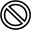y  = (5x - 4y)/y , find the value of y if 6y = 2.

5

10

2

4

5

Explanation:

If we substitute 6 in for x in the given equation and set our answer to 2, we can solve for y algebraically. 30 minus 4y divided by y equals 2 -->2y =30 -4y --> 6y =30 --> y=5.  We could also work from the answers and substitute each answer in and solve.

### Example Question #1001 : Algebra

Simplify the following expression: x3 - 4(x2 + 3) + 15

x3 – 4x2 + 3

x5 + 3

x3 – 12x2 + 15

x3 – 3x2 + 15

x3 – 4x2 + 3

Explanation:

To simplify this expression, you must combine like terms. You should first use the distributive property and multiply -4 by x2 and -4 by 3.

x3 - 4x2 -12 + 15

You can then add -12 and 15, which equals 3.

You now have x3 - 4x2 + 3 and are finished. Just a reminder that x3 and 4x2 are not like terms as the x’s have different exponents.

### Example Question #763 : Algebra

Evaluate: (2x + 4)(x2 – 2x + 4)

2x3 + 16

2x3 + 8x2 – 16x – 16

2x3 – 8x2 + 16x + 16

4x2 + 16x + 16

2x3 – 4x2 + 8x

2x3 + 16

Explanation:

Multiply each term of the first factor by each term of the second factor and then combine like terms.

(2x + 4)(x2 – 2x + 4) = 2x3 – 4x2 + 8x  +  4x2 – 8x + 16 = 2x3 + 16

### Example Question #1 : How To Simplify An Expression

Which of the following is equivalent to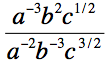?

ab/c

a2/(b5c)

abc

ab5c

b5/(ac)

b5/(ac)

Explanation:

First, we can use the property of exponents that xy/xz = xy–z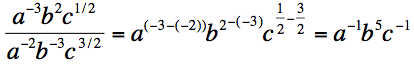Then we can use the property of exponents that states x–y = 1/xy

a–1b5c–1 = b5/ac

### Example Question #2 : Simplifying Expressions

Solve for x: 2y/3b = 5x/7a

15b/14ay

6ab/7y

14ay/15b

5by/3a

7ab/6y

14ay/15b

Explanation:

Cross multiply to get 14ay = 15bx, then divide by 15b to get x by itself.

### Example Question #1 : How To Simplify An Expression

Three consecutive positive integers are added together. If the largest of the three numbers is m, find the sum of the three numbers in terms of m.

3m

3m – 3

3m + 6

3m + 3

3m – 6

3m – 3

Explanation:

Three consecutive positive integers are added together.  If the largest of the three numbers is m, find the sum of the three numbers in terms of m.

If m is the largest of three consecutive positive integers, then the integers must be:

m – 2, m – 1, and m, where m > 2.

The sum of these three numbers is:

m - 2 + m – 1 + m = 3m – 3

### Example Question #1 : Simplifying Expressions

Sophie travels f miles in g hours.  She must drive another 30 miles at the same rate.  Find the total number of hours, in terms of f and g, that the trip will take.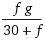g + f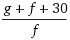g + f + 30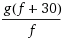Explanation:

Using d = rt, we know that first part of the trip can be represented by f = rg.  The second part of the trip can be represented by 30 = rx, where x is some unknown number of hours.  Note that the rate r is in both equations because Sophie is traveling at the same rate as mentioned in the problem.

Solve each equation for the time (g in equation 1, x in equation 2).

g = f/r

x = 30/r

The total time is the sum of these two times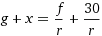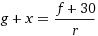Note that, from equation 1, r = f/g, so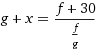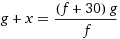=### Example Question #1 : Simplifying Expressions

If ab = 10 and bc = 15, then what is the value of (c – a)/(a + 2b + c)?

150

1/5

3/2

5

2/3

1/5

Explanation:

a + b = 10

b + c = 15

------------

a + b + b + c = 10 + 15

a + 2b + c = 25 (this is the denominator of the answer)

Subtract the two equations:

b + c = 15

a + b = 10

------------

b + c – (a + b) = 15 – 10

c – a = 5 (this is the numerator of the answer)

5/25 = 1/5

← Previous 1 3 4 5 6 7 8

### All SAT Math Resources